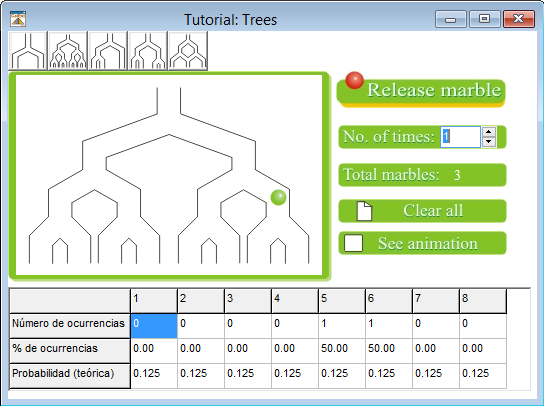## CalEst Environment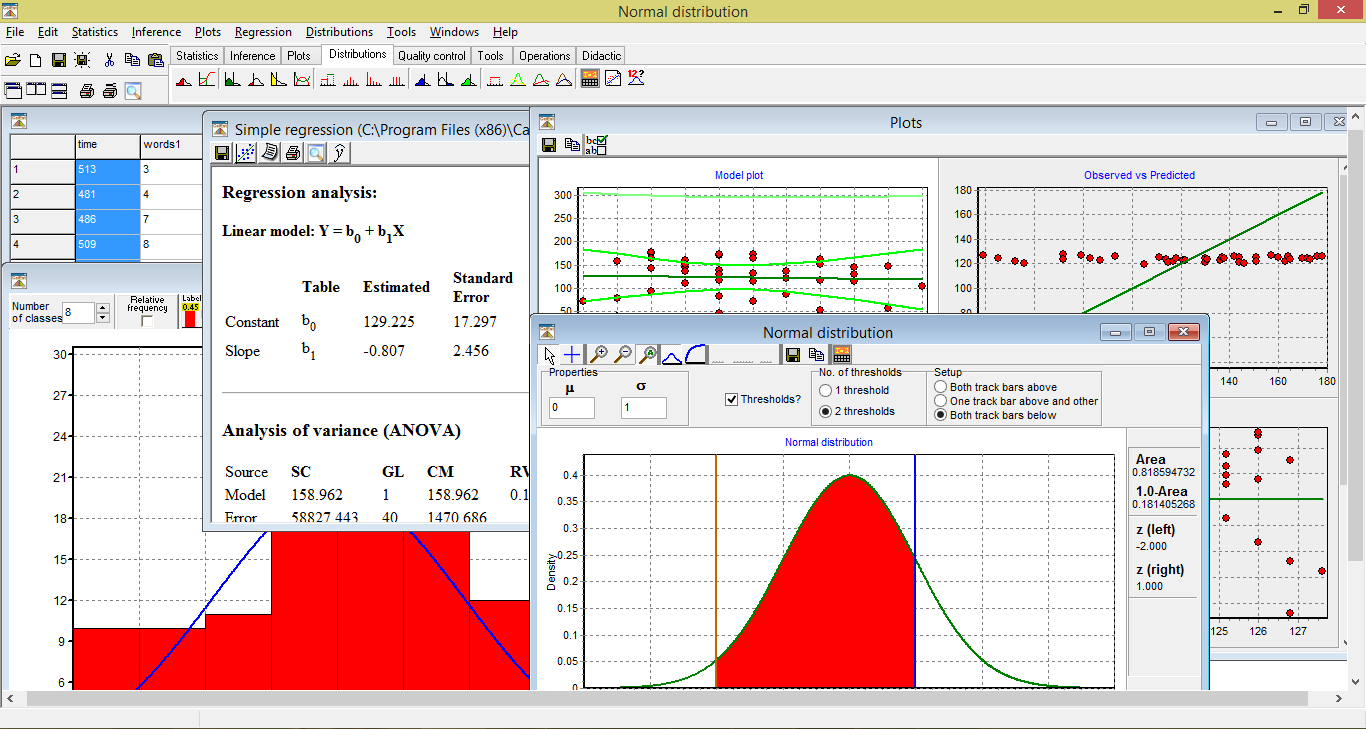## CalEst Features

### Statistics

• Measurements of central tendency (mean, median, mode, standard deviation, percentiles, variance, skewness, kurtosis, geometric mean, harmonic mean, etc.)
• Process capability
• Control charts (x-R y x-S)
• Six Sigma (normal distribution)

### Measurements (options)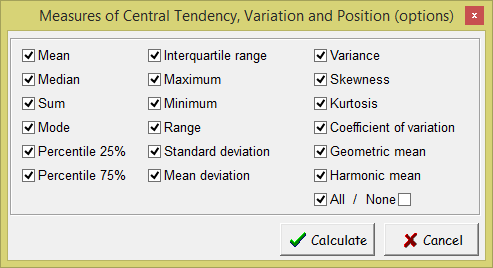### Design of experiments

• 2k Factorial
• Plackett-Burman
• Central composite
• Box-Behnken
• General Factorial

### Design of experiments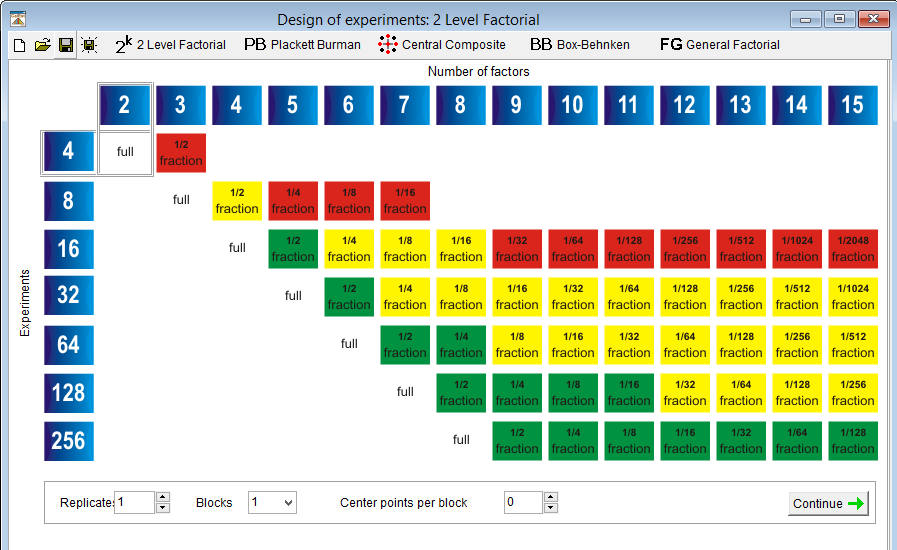### Inference

• Hypothesis test
• One mean (t, z, Chi-squared)
• Two means (independent, paired; z, t, F)
• Proportions
• Variance (Chi-squared, F)
• Non parametric (K-S test, sign test)
• Confidence intervals
• ANOVA
• Chi-squared test (bondad de ajuste, tables)

### Hypothesis test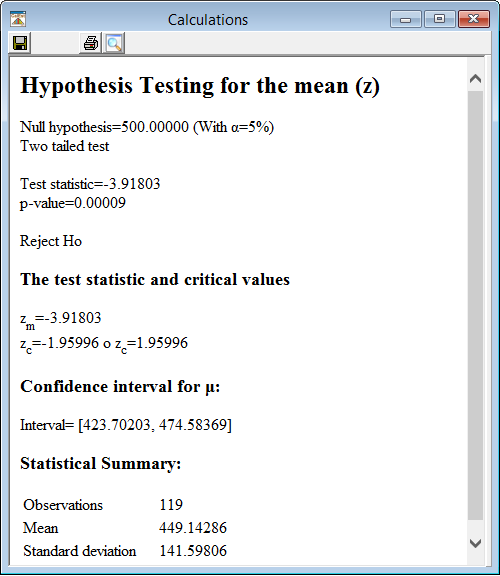### Graphics

• Histogram
• Polygon-cumulative frequency
• Frequency Polygons
• Empirical distribution FDA
• Histogram + Polygon
• Pie chart
• Dot plot
• Stem-and-Leaf plot
• Box plot
• Dispersion plot
• Frequency table

### Histogram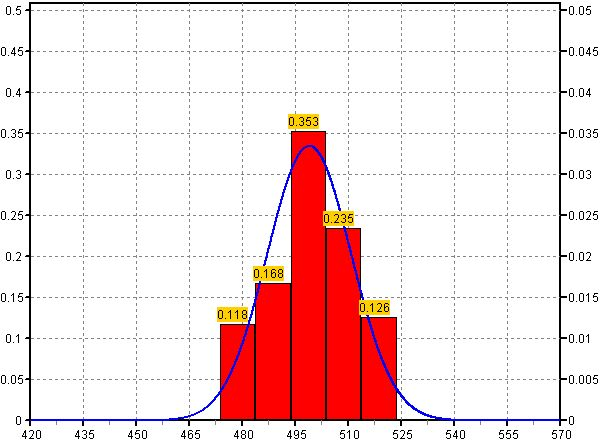• Simple
• Multiple
• Polynomial

### Regression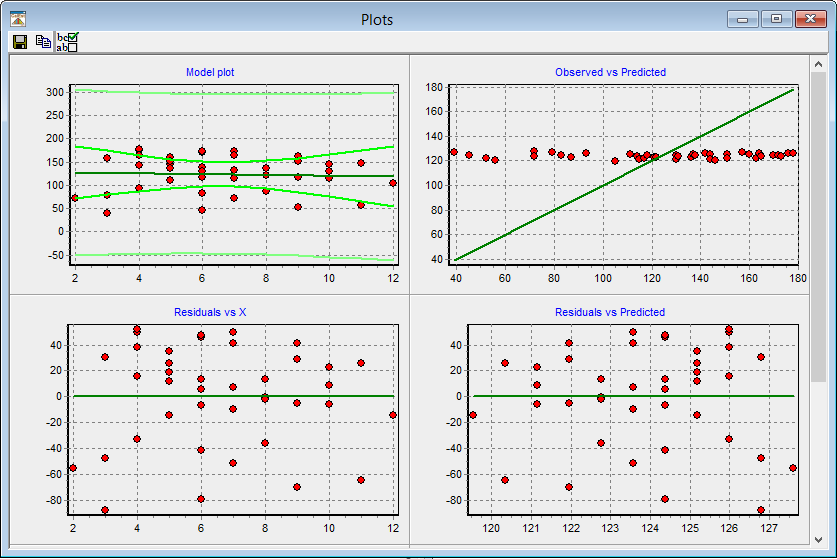### Distributions (density and cumulative)

• Normal
• Weibull
• Gamma
• Exponential
• Beta
• t-Student
• Chi-squared
• F
• Lognormal
• Logistic
• Extreme value
• Uniform (continuous and discrete)
• Bernoulli
• Binomial
• Poisson
• Calculator
• Probability paper

### Normal distribution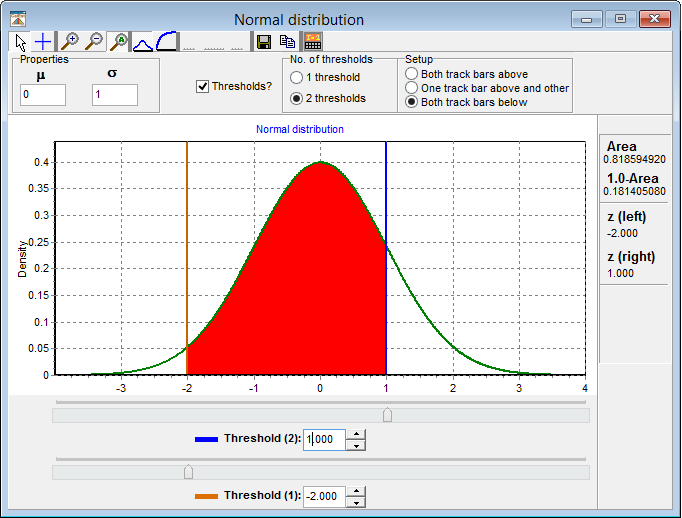### Tools

• Sort data (from low to high, from high to low, shuffling data)
• Operations between data columns (normalization, logarithmic, exponential, inverse, etc.)
• Random Number Generator
• Number generator following a function
• Counting techniques

### Tutorials

• Probability games
• Toss
• A die
• Mission to Mars (robot, artificial intelligence)
• Two dice
• Coin and die
• Toss (binomial distribution)
• Trees (marbles)
• Noise and image processing
• Hypothesis test
• Theorem of central limit(dice)
• Motivation (context)
• Iris identification
• Quality Control
• Catapult
• Helicopter

### Tutorial: Trees